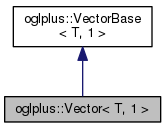OGLplus (0.52.0) a C++ wrapper for OpenGLoglplus::Vector< T, 1 > Class Template Reference

`#include </home/chochlik/devel/oglplus/include/oglplus/math/vector.hpp>`

Inheritance diagram for oglplus::Vector< T, 1 >:[legend]
Collaboration diagram for oglplus::Vector< T, 1 >:[legend]

Public Member Functions

TData (void)
Pointer to the components of this vector.

const TData (void) const
Pointer to the components of this vector.

T At (std::size_t i) const

T At (std::size_t i, T fallback) const
Access to the i-th component of this vector with a fallback. More...

Toperator[] (std::size_t i)

const Toperator[] (std::size_t i) const

Adds `v` to this vector.

void Subtract (const VectorBase &v)
Subtracts `v` from this vector.

void Multiply (T v)
Multiplies this vector by a scalar value.

void Multiply (const VectorBase &that)
Multiplies the elements of this and that vector.

void Divide (T v)
Divides this vector by a scalar value.

void Divide (const VectorBase &that)
Divides the elements of this and that vector.

T Length (void) const
Returns the lenght of this vector.

bool IsNormal (T eps=T(0)) const
Returns true if the vector is normal.

void Normalize (void)
Normalizes this vector.

Static Public Member Functions

static std::size_t Size (void)
The size (the number of components) of this vector.

static T DotProduct (const VectorBase &a, const VectorBase &b)
Computes the dot product of vectors `a` and `b`.

Detailed Description

template<typename T> class oglplus::Vector< T, 1 >

.file oglplus/math/vector_1.ipp .brief Specialization of Vector for 1D vectors

Member Function Documentation

 T oglplus::VectorBase< T, N >::At ( std::size_t i ) const
inherited

Precondition
(i < Size())
 T oglplus::VectorBase< T, N >::At ( std::size_t i, T fallback ) const
inherited

Similar to At(i), but returns `fallback` if `i` is greater than or equal to Size().

 T& oglplus::VectorBase< T, N >::operator[] ( std::size_t i )
inherited

Precondition
(i < Size())
 const T& oglplus::VectorBase< T, N >::operator[] ( std::size_t i ) const
inherited